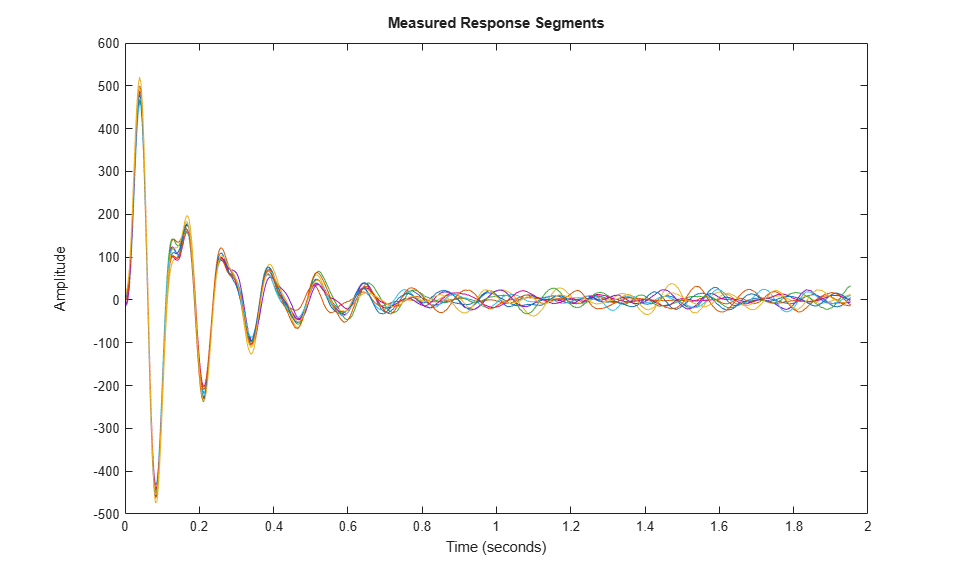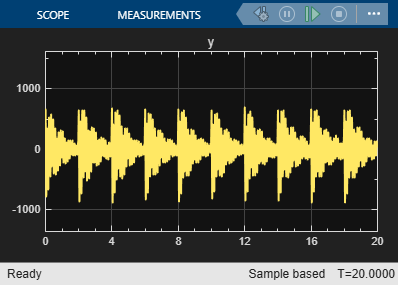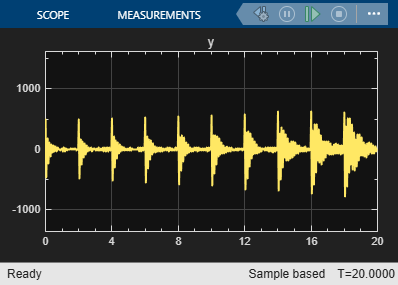## Fault Detection Using Data Based Models

This example shows how to use a data-based modeling approach for fault detection.

### Introduction

Early detection and isolation of anomalies in a machine's operation can help to reduce accidents, reduce downtime and thus save operational costs. The approach involves processing live measurements from a system's operation to flag any unexpected behavior that would point towards a newly developed fault.

This example explores the following fault diagnosis aspects:

1. Detection of abnormal system behavior by residual analysis

2. Detection of deterioration by building models of a damaged system

3. Tracking system changes using online adaptation of model parameters

### Identifying a Dynamic Model of System Behavior

In a model based approach to detection, a dynamic model of the concerned system is first built using measured input and output data. A good model is able to accurately predict the response of the system for a certain future time horizon. When the prediction is not good, the residuals may be large and could contain correlations. These aspects are exploited to detect the incidence of failure.

Consider a building subject to impacts and vibrations. The source of vibrations can be different types of stimuli depending upon the system such as wind gusts, contact with running engines and turbines, or ground vibrations. The impacts are a result of impulsive bump tests on the system that are added to excite the system sufficiently. Simulink model `idMechanicalSystem.slx` is a simple example of such a structure. The excitation comes from periodic bumps as well as ground vibrations modeled by filtered white noise. The output of the system is collected by a sensor that is subject to measurement noise. The model is able to simulate various scenarios involving the structure in a healthy or a damaged state.

```sysA = 'pdmMechanicalSystem'; open_system(sysA) % Set the model in the healthy mode of operation set_param([sysA,'/Mechanical System'],'OverrideUsingVariant','Normal') % Simulate the system and log the response data sim(sysA) ynormal = logsout.getElement('y').Values; ```The input signal was not measured; all we have recorded is the response `ynormal`. Hence we build a dynamic model of the system using "blind identification" techniques. In particular, we build an ARMA model of the recorded signal as a representation of the system. This approach works when the input signal is assumed to be (filtered) white noise. Since the data is subject to periodic bumps, we split the data into several pieces each starting at the incidence of a bump. This way, each data segment contains the response to one bump plus random excitations - a situation that can be captured using a time series model, where the effect of the bump is attributed to suitable initial conditions.

```Ts = 1/256; % data sample time nr = 10; % number of bumps in the signal N = 512; % length of data between bumps znormal = cell(nr,1); for ct = 1:nr ysegment = ynormal.Data((ct-1)*N+(1:500)); z = iddata(ysegment,[],Ts); znormal{ct} = z; % each segment has only one bump end plot(znormal{:}) % plot a sampling of the recorded segments title('Measured Response Segments') ```Split the data into estimation and validation pieces.

```ze = merge(znormal{1:5}); zv = merge(znormal{6:10}); ```

Estimate a 7th order time-series model in state-space form using the `ssest()` command. The model order was chosen by cross validation (checking the fit to validation data) and residual analysis (checking that residuals are uncorrelated).

```nx = 7; model = ssest(ze, nx, 'form', 'canonical', 'Ts', Ts); present(model) % view model equations with parameter uncertainty ```
``` model = Discrete-time identified state-space model: x(t+Ts) = A x(t) + K e(t) y(t) = C x(t) + e(t) A = x1 x2 x3 x1 0 1 0 x2 0 0 1 x3 0 0 0 x4 0 0 0 x5 0 0 0 x6 0 0 0 x7 0.5548 +/- 0.04606 -2.713 +/- 0.2198 5.885 +/- 0.4495 x4 x5 x6 x1 0 0 0 x2 0 0 0 x3 1 0 0 x4 0 1 0 x5 0 0 1 x6 0 0 0 x7 -8.27 +/- 0.5121 9.234 +/- 0.3513 -7.956 +/- 0.1408 x7 x1 0 x2 0 x3 0 x4 0 x5 0 x6 1 x7 4.263 +/- 0.02599 C = x1 x2 x3 x4 x5 x6 x7 y1 1 0 0 0 0 0 0 K = y1 x1 1.025 +/- 0.01401 x2 1.444 +/- 0.0131 x3 1.907 +/- 0.01271 x4 2.385 +/- 0.01203 x5 2.857 +/- 0.01456 x6 3.26 +/- 0.0222 x7 3.552 +/- 0.0336 Sample time: 0.0039062 seconds Parameterization: CANONICAL form with indices: 7. Disturbance component: estimate Number of free coefficients: 14 Use "idssdata", "getpvec", "getcov" for parameters and their uncertainties. Status: Termination condition: Near (local) minimum, (norm(g) < tol).. Number of iterations: 7, Number of function evaluations: 15 Estimated using SSEST on time domain data "ze". Fit to estimation data: [99.07 99.04 99.15 99.05 99.04]% (prediction focus) FPE: 0.6242, MSE: [0.5974 0.6531 0.5991 0.5871 0.6496] More information in model's "Report" property. ```

The model display shows relatively small uncertainty in parameter estimates. We can confirm the reliability by computing the 1-sd (99.73%) confidence bound on the estimated spectrum of the measured signal.

```h = spectrumplot(model); showConfidence(h, 3) ```The confidence region is small, although there is about 30% uncertainty in the response at lower frequencies. The next step in validation is to see how well the model predicts the responses in the validation dataset `zv`. We use a 25-step ahead prediction horizon.

```compare(zv, model, 25) % Validation against one dataset ```The plot shows that the model is able to predict the response in the first experiment of the validation dataset 25 time steps (= 0.1 sec) in future with > 85% accuracy. To view the fit to other experiments in the dataset, use the right-click context menu of the plot axes.

The final step in validating the model is to analyze the residuals generated by it. For a good model, these residuals should be white, i.e., show statistically insignificant correlations for non-zero lags:

```resid(model, zv) ```The residuals are mostly uncorrelated at nonzero lags. Having derived a model of the normal behavior we move on to investigate how the model can be used to detect faults.

### Fault Detection by Residual Analysis Using Model of Healthy State

Fault detection is tagging of unwanted or unexpected changes in observations of the system. A fault causes changes in the system dynamics owing either to gradual wear and tear or sudden changes caused by sensor failure or broken parts. When a fault appears, the model obtained under normal working conditions is unable to predict the observed responses. This causes the difference between the measured and predicted response (the residuals) to increase. Such deviations are usually flagged by a large squared-sum-of-residuals or by presence of correlations.

Put the Simulink model in the damaged-system variant and simulate. We use a single bump as input since the residual test needs white input with possibly a transient owing to initial conditions.

```set_param([sysA,'/Mechanical System'],'OverrideUsingVariant','DamagedSystem'); set_param([sysA,'/Pulse'],'Period','5120') % to force only one bump sim(sysA) y = logsout.getElement('y').Values; ``````resid(model, y.Data) set_param([sysA,'/Pulse'],'Period','512') % restore original ```The residuals are now larger and show correlations at non-zero lags. This is the basic idea behind detection of faults - creating a residual metric and observing how it changes with each new set of measurements. What is used here is a simple residual based on 1-step prediction error. In practice, more advanced residuals are generated that are tailor-made to the application needs.

### Fault Detection Using Models of Normal and Deteriorated State

A more detailed approach to fault detection is to also identify a model of the faulty (damaged) state of the system. We can then analyze which model is more likely to explain the live measurements from the system. This arrangement can be generalized to models for various types of faults and thus used for not just detecting the fault but also identifying which one ("isolation"). In this example, we take the following approach:

1. We collect data with system operating in the normal (healthy) and a known wear-and-tear induced end-of-life state.

2. We identify a dynamic model representing the behavior in each state.

3. We use a data clustering approach to draw a clear distinction between these states.

4. For fault detection, we collect data from the running machine and identify a model of its behavior. We then predict which state (normal or damaged) is most likely to explain the observed behavior.

We have already simulated the system in its normal operation mode. We now simulate the model `idMechanicalSystem` in the "end of life" mode. This is the scenario where the system has already deteriorated to its final state of permissible operation.

```set_param([sysA,'/Mechanical System'],'OverrideUsingVariant','DamagedSystem'); sim(sysA) y = logsout.getElement('y').Values; zfault = cell(nr,1); for ct = 1:nr z = iddata(y.Data((ct-1)*N+(1:500)),[],Ts); zfault{ct} = z; end ```We now create a set of models, one for each data segment. As before we build 7th order time series models in state-space form. Turn off covariance computation for speed.

```mNormal = cell(nr,1); mFault = cell(nr, 1); nx = order(model); opt = ssestOptions('EstimateCovariance',0); for ct = 1:nr mNormal{ct} = ssest(znormal{ct}, nx, 'form', 'canonical', 'Ts', Ts, opt); mFault{ct} = ssest(zfault{ct}, nx, 'form', 'canonical', 'Ts', Ts, opt); end ```

Verify that the models `mFault` are a good representation of the faulty mode of operation:

```compare(merge(zfault{:}), mFault{:}, 25) ```Normal and faulty estimated spectra are plotted below.

```Color1 = 'k'; Color2 = 'r'; ModelSet1 = cat(2,mNormal,repmat({Color1},[nr, 1]))'; ModelSet2 = cat(2,mFault,repmat({Color2},[nr, 1]))'; spectrum(ModelSet1{:},ModelSet2{:}) axis([1 1000 -45 40]) title('Output Spectra (black: normal, red: faulty)') ```The spectrum plot shows the difference: the damaged mode has its primary resonances amplified but the spectra are otherwise overlapping. Next, we create a way to quantitatively distinguish between the normal and the faulty state. We can use data clustering and classification approaches such as:

• Fuzzy C-Means Clustering. See `fcm()` in Fuzzy Logic Toolbox.

• Support Vector Machine Classifier. See `fitcsvm ()` in Statistics and Machine Learning Toolbox.

• Self-organizing Maps. See `selforgmap()` in Deep Learning Toolbox.

In this example, we use the Support Vector Machine classification technique. The clustering of information from the two types of models (`mNormal` and `mFault`) can be based on different kinds of information that these models can provide such as the locations of their poles and zeroes, their locations of peak resonances or their list of parameters. Here, we classify the modes by the pole locations corresponding to the two resonances. For clustering, we tag the poles of the healthy state models with 'good' and the poles of the faulty state models with 'faulty'.

```ModelTags = cell(nr*2,1); % nr is number of data segments ModelTags(1:nr) = {'good'}; ModelTags(nr+1:end) = {'faulty'}; ParData = zeros(nr*2,4); plist = @(p)[real(p(1)),imag(p(1)),real(p(3)),imag(p(3))]; % poles of dominant resonances for ct = 1:nr ParData(ct,:) = plist(esort(pole(mNormal{ct}))); ParData(nr+ct,:) = plist(esort(pole(mFault{ct}))); end cl = fitcsvm(ParData,ModelTags,'KernelFunction','rbf', ... 'BoxConstraint',Inf,'ClassNames',{'good', 'faulty'}); cl.ConvergenceInfo.Converged ```
```ans = logical 1 ```

`cl` is an SVM classifier that separates the training data `ParData` into good and faulty regions. Using the `predict` method of this classifier one can assign an input nx-by-1 vector to one of the two regions.

Now we can test the classifier for its prediction (normal vs damaged) collect data batches from a system whose parameters are changing in a manner that it goes from being healthy (mode = 'Normal') to being fully damaged (mode = 'DamagedSystem') in a continuous manner. To simulate this scenario, we put the model in 'DeterioratingSystem' mode.

```set_param([sysA,'/Mechanical System'],'OverrideUsingVariant','DeterioratingSystem'); sim(sysA) ytv = logsout.getElement('y').Values; ytv = squeeze(ytv.Data); PredictedMode = cell(nr,1); for ct = 1:nr zSegment = iddata(ytv((ct-1)*512+(1:500)),[],Ts); mSegment = ssest(zSegment, nx, 'form', 'canonical', 'Ts', Ts); PredictedMode(ct) = predict(cl, plist(esort(pole(mSegment)))); end I = strcmp(PredictedMode,'good'); Tags = ones(nr,1); Tags(~I) = -1; t = (0:5120)'*Ts; % simulation time Time = t(1:512:end-1); plot(Time(I),Tags(I),'g*',Time(~I),Tags(~I),'r*','MarkerSize',12) grid on axis([0 20 -2 2]) title('Green: Normal, Red: Faulty state') xlabel('Data evaluation time') ylabel('Prediction') ```The plot shows that the classifier predicts the behavior up to about the mid-point to be normal and in a state of fault thereafter.

### Fault Detection by Online Adaptation of Model Parameters

The preceding analysis used batches of data collected at different times during the operation of the system. An alternative, often more convenient, way of monitoring the health of the system is to create an adaptive model of its behavior. The new measurements are processed continuously and are used to update the parameters of a model in a recursive fashion. The effect of wear and tear or a fault is indicated by a change in the model parameter values.

Consider the wear-and-tear scenario again. As the system ages, there is a greater "rattling" which manifests itself as excitation of several resonant modes as well as a rise in the system's peak response. This scenario is described in model `idDeterioratingSystemEstimation` which is same as the 'DeterioratingSystem' mode of `idMechanicalSystem` except that the impulsive bumps that were added for offline identification are not present. The response of the system is passed to a "Recursive Polynomial Model Estimator" block which has been configured to estimate the parameters of an ARMA model structure. The actual system starts in a healthy state but deteriorates to end-of-life conditions over a time span of 200 seconds.

```initial_model = translatecov(@(x)idpoly(x),model); sysB = 'pdmDeterioratingSystemEstimation'; open_system(sysB); ```The "ARMA model" block has been initialized using the parameters and covariance data from the estimated model of normal behavior derived in the previous section after conversion to polynomial (ARMA) format. The `translatecov()` function is used so that the parameter covariance data is also converted. The block uses a "Forgetting factor" algorithm with the forgetting factor set to slightly less than 1 to update the parameters at each sampling instant. The choice of forgetting factor influences how rapidly the system updates. A small value means that the updates will have high variance while a large value will make it harder for the estimator to adapt to fast changes.

The model parameters estimate is used to update the output spectrum and its 3-sd confidence region. The system will have clearly changed when the spectrum's confidence region does not overlap that of the healthy system at frequencies of interest. A fault detection threshold is shown using a black line in the plot marking the maximum allowed gains at certain frequencies. As changes in the system accumulate, the spectrum drifts across this line. This serves as a visual indicator of a fault which can be used to call for repairs in real-life systems.

Run the simulation and watch the spectrum plot as it updates.

```sim(sysB) ```The running estimates of model parameters are also used to compute the system pole locations which are then fed into the SVM classifier to predict if the system is in the "good" or "fault" state. This decision is also displayed on the plot. When the normalized score of prediction is less than .3, the decision is considered tentative (close to the boundary of distinction). See the script `pdmARMASpectrumPlot.m` for details on how the running estimate of spectrum and classifier prediction is computed.

It is possible to implement the adaptive estimation and plotting procedure outside Simulink using the `recursiveARMA()` function. Both the "Recursive Polynomial Model Estimator" block as well as the `recursiveARMA()` function support code generation for deployment purposes.

The classification scheme can be generalized to the case where there are several known modes of failure. For this we will need multi-group classifiers where a mode refers to a certain type of failure. These aspects are not explored in this example.

### Conclusions

This example showed how system identification schemes combined with data clustering and classification approaches can assist in detection and isolation of faults. Both sequential batch analysis as well as online adaptation schemes were discussed. A model of ARMA structure of the measured output signal was identified. A similar approach can be adopted in situations where one has access to both input and output signals, and would like to employ other types of model structures such as the State-space or Box-Jenkins polynomial models.

In this example, we found that:

1. Correlations in residuals based on a model of normal operation can indicate onset of failure.

2. Gradually worsening faults can be detected by employing a continuously adapting model of the system behavior. Preset thresholds on a model's characteristics such as bounds on its output spectrum can help visualize the onset and progression of failures.

3. When the source of a fault needs to be isolated, a viable approach is to create separate models of concerned failure modes beforehand. Then a classification approach can be used to assign the predicted state of the system to one of these modes.